﻿Two proportion z test

# Two Sample proportion Z-Test

Hypotheses
H0: p1 = p2
H1: p1 < > p2
Test statisticNormal distribution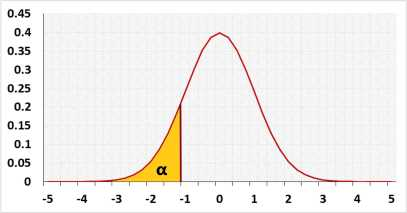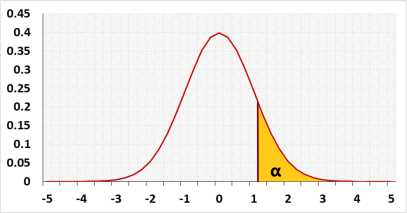Test parameters
The default is two tailed test.
example digits=2: (0.001234 => 0.0012)
Significant level (0-1), maximum chance allowed rejecting H0 while H0 is correct (Type1 Error)
continuity correction from binomial distribution to the normal distribution, the default is True.
The test is expected to identify this effect. If one exists, H0 will be rejected. h effects examples( 0.2 - small effect, 0.5 - medium effect, 0.8 - large effect). more
Expected difference, usually zero
You may calculate h based on the expected proportions:

### Calcualate h effect size

expected sample1's proportion, the power is not sensitive to this value, if you leave it empty the tool will use the estimte p: (x1+x2)/(n1+n2)
expected sample2's proportion to be able to detect

Sample data

The population's name
sample1's proportion or number of successes
Sample size
The population's name
sample2's proportion or number of successes
Sample size

## Information

Target: To check if the difference between the probability of succees (p) of two groups (populations) is significant, using sample data

## Assumptions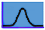Binomical distribution - the probability for event within each group is identicalExpected difference d between the populations's average is known
Required Sample Data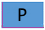p1, p2 the sample probabilities or x1, x2 the number of successes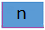n1,n2 - Sample size of group1 and group2

## R Code

The following R code should produce the same results:

The prop.test uses the Chi-squared statistic with one degree of freedom, which is exactly as using the Z test: z22(1)